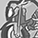# QlikView App Dev

Discussion Board for collaboration related to QlikView App Development.

Announcements
QlikWorld 2023, a live, in-person thrill ride. Save \$300 before February 6: REGISTER NOW!
cancel
Showing results for
Did you mean:Creator

## Expression for calculating the mean of a number

HI Folks,

I have the below expression and I want to get the mean percentage of the number

So for example for the % assessed of those presenting it’ll be

=(Count(NEWKEYID)-Count(PatientsNotAssessed_ID))/count(Hospital)

So in other words thats 6946/28 which gives me a mean national value of 248 approx.

So to get the mean percentage of the number is it 248/6946 *100 =3.5%??

What would the expression be then?  Any ideas?

4 RepliesMVP

May be try this

`(((Count(NEWKEYID)-Count(PatientsNotAssessed_ID))/count(Hospital))/(Count(NEWKEYID)-Count(PatientsNotAssessed_ID))) * 100`

Or Simplify it to this

`(1 - (Count(Hospital)/(Count(NEWKEYID)-Count(PatientsNotAssessed_ID)))) * 100`MVP

Or simplify it to this

`1/Count(Hospital) * 100`Creator
Author
Thanks Stalwar

I think Im calculating the mean percentage wrong so I have 28 hospitals with 6946 patients.
The mean number for this is 6946/28 =248.

But the mean percentage of this number should be 248/(highest number on that list) which in that case is 861 and I *100.

Is that the way i should be calculating the mean % so 248/861*100 is 28%? ...is that correct?apologies probably basic maths!MVP
I am not sure I follow. Can you share a sample to check it out?Community Browser